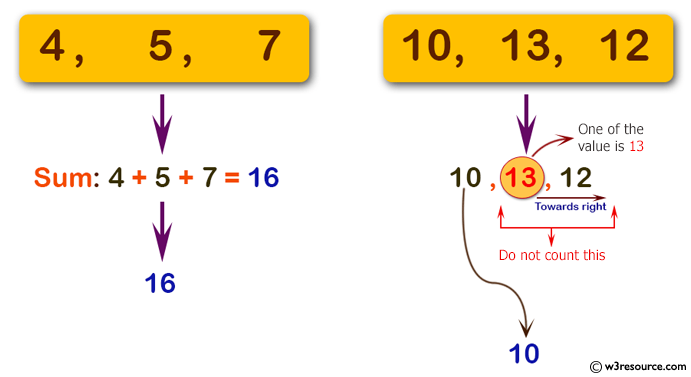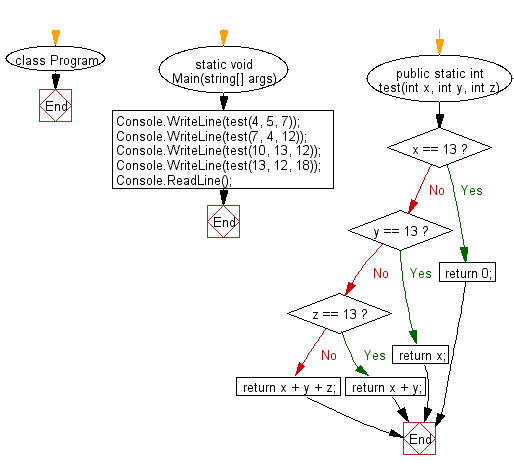﻿ C# - Sum the three integers, excluding 13 if any of them is# C# Sharp Basic Algorithm Exercises: Compute the sum of the three integers. If one of the values is 13 then do not count it and its right towards the sum

## C# Sharp Basic Algorithm: Exercise-56 with Solution

Write a C# Sharp program to compute the sum of the three integers. If one of the values is 13 then do not count it and its right towards the sum.

Pictorial Presentation:Sample Solution:-

C# Sharp Code:

``````using System;
using System.Linq;
namespace exercises
{
class Program
{
static void Main(string[] args)
{
Console.WriteLine(test(4, 5, 7));
Console.WriteLine(test(7, 4, 12));
Console.WriteLine(test(10, 13, 12));
Console.WriteLine(test(13, 12, 18));
}

public static int test(int x, int y, int z)
{
if (x == 13) return 0;
if (y == 13) return x;
if (z == 13) return x + y;
return x + y + z;
}
}
}
```
```

Sample Output:

```16
23
10
0```

Flowchart:C# Sharp Code Editor:

Improve this sample solution and post your code through Disqus

What is the difficulty level of this exercise?

Test your Programming skills with w3resource's quiz.

﻿